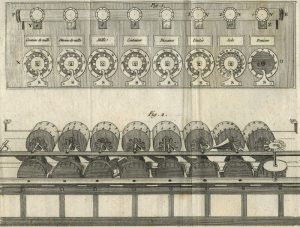Mathematics

# Pascaline

Pascaline was the first mechanical calculator that worked through a series of wheels and gears. It was initially known as the "arithmetic machine", then called the "Pascaline wheel", and finally got its name as Pascaline. This mathematical apparatus had the ability to add, subtract, although it did not do so directly, the machine was also capable of multiplying and dividing by means of subtractions or additions in a repetitive manner.Related topics

Abacus

## What is Pascaline?

Pascaline was the first calculator invented in the world, it worked by means of a series of wheels and different gears, which could add, subtract, divide and multiply by subtractions and additions in a repetitive way.

## Characteristics of the pascaline

• It could only do addition and subtraction, with numbers entered by manipulating its dials.
• The Pascaline is the size of a shoebox and that’s why it can be easily carried.
• It has eight different windows at the top.
• Inside each of the windows, a small drum can be seen with the digits of the result.
• Each drum is made up of two different number rows.
• In front of the windows there are eight adjustment mechanisms.
• Depending on the positional value of the quantity to be added, the wheels will move according to the positions as they correspond to the value of the corresponding digit.
• Each wheel on the top of the Pascaline has an axle that has a horizontal crown gear. The gear is transmitting the wheel rotation to a vertical crown gear.
• The subtraction could not be made by turning the machine wheel in the opposite direction but had to be made by an indirect method known as nines complements, which is made by turning the wheels in the same direction.
• The first Pascaline could only use 5-digit numbers, but then Pascal developed 6-digit and 8-digit versions.

## History

Informatics history goes back to ancient times. The most remote example is the abacus, an adding instrument still used in some parts of Japan and Eastern Europe. French mathematician and philosopher Blaise Pascal invented the world’s first mechanical calculator in 1642 to help his father, who was the area’s tax inspector. The machine worked perfectly, was able to carry the numbers from the column of units to the column of tens by means of a ratchet mechanism and was fully functional. Blaise decided to call it Pascaline. Gottfried Leibniz worked on perfecting the Pascal adding machine and attempted to improve it so that it would be able to multiply and divide by achieving this goal through the placement of a mechanical device called the Leibniz cylinder. After having perfected this machine, Leibniz focused his efforts on creating a method that would allow the decimal system to be converted into a binary-based system. The first use of Pascaline was in the French farm, where Pascal’s father worked.

## Who invented the pascaline

Pascaline was invented by Blaise Pascal in 1642. It was the son of a civil servant whose job was to collect taxes. Pascal, who occasionally helped his father to write his official reports, wondered how to help his father in the different arithmetic operations in which large numbers had to be added.

## How it works

Pascaline had the shape of a shoe box and was low and somewhat elongated. On the inside, there were a series of sprockets that were connected to each other, thus forming a transmission chain, so that when a wheel turned completely on its axle, it advanced one degree to the next. These different wheels that were inside the Pascaline had as function to represent the decimal system of numeration. Each wheel consisted of ten steps, so it was also marked with numbers ranging from 9 to 0. In total it consisted of eight wheels, six of them were used to represent whole numbers and two more wheels, at the far left, to represent decimal numbers. With this arrangement, whole numbers between 0’01 and 999.999’99 could be handled. By means of a crank, the toothed wheels could turn to achieve adding or subtracting in this way. If a number needed to be subtracted, the crank had to be operated in the opposite direction.

Written by Gabriela Briceño V.(5 votos, promedio: 4.60 de 5)Loading...